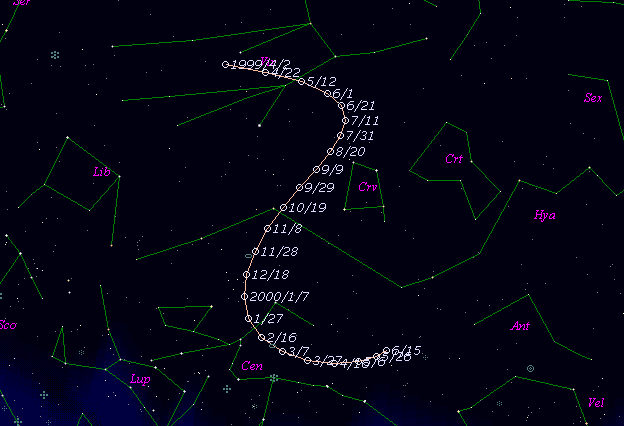# \$B%j%K%"WB@1(B

C/1999 G1 ( LINEAR )###\$B%W%m%U%#!<%k(B

 \$BH/8+F|(B 1999\$BG/(B 4\$B7n(B 7\$BF|(B \$BH/8+8wEY(B 18.9\$BEy(B \$BH/8+ Lincoln Laboratory Near-Earth Asteroid Research project

###\$B###\$B50F;MWAG(B

```    Orbital elements by K. Muraoka,
from 42 observations    1999 Apr. 8 to May 13,
m.e. =  +/- 0.92 arc seconds.

T  =  1998 July 31.9308  TT
Peri. =  136.0145
Node  =   23.4840   (2000.0)
Incl. =   76.3145
q  =    4.040547  AU
e  =    0.845170
a  =   26.096670  AU
n  =    0.0073931
P  =  133.32     years
```

###\$B@1?^(B###\$B8wEYJQ2=(B

```        m1 = 8.0 + 5 log\$B&\$(B + 10.0 log r
```##### \$B50F;MWAG\$OB<2,7r<#;a\$N7W;;\$K\$h\$k\$b\$N\$G\$9!#(B \$B@1?^\$O(B StellaNavigator Ver.2.0 for Windows (\$B%"%9%H%m%"!<%D(B \$BJTCx(B / \$B%"%9%-!<=PHG6I4)(B) \$B\$G:n@.\$7\$?\$b\$N\$G\$9!#(B \$B8wEY%0%i%U\$O(BComet for Windows\$B\$G:n@.\$7\$?\$b\$N\$G\$9!#(B